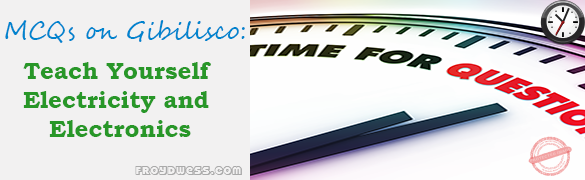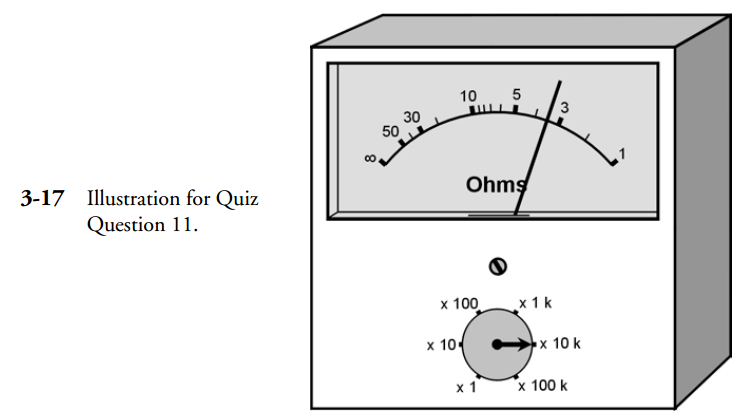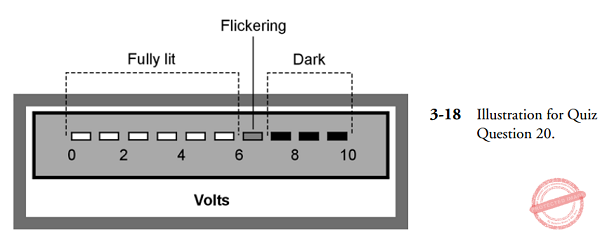# Measuring Devices – Gibilisco MCQs in Electronics

(Last Updated On: December 8, 2017)This is the Multiple Choice Questions (MCQs) in Chapter 3: Measuring Devices from the book Teach Yourself Electricity and Electronics, 5th edition by Stan Gibilisco. If you are looking for a reviewer in Electronics Engineering this will definitely help you before taking the Board Exam.

### Start Practice Exam Test Questions

1. The force between two electrically charged objects is called:

• A. Electromagnetic deflection.
• B. Electrostatic force.
• C. Magnetic force.
• D. Electroscopic force.

2. The change in the direction of a compass needle, when a current-carrying wire is brought near, is:

• A. Electromagnetic deflection.
• B. Electrostatic force.
• C. Magnetic force.
• D. Electroscopic force.

3. Suppose a certain current in a galvanometer causes the needle to deflect 20 degrees, and then this current is doubled. The needle deflection:

• A. Will decrease.
• B. Will stay the same.
• C. Will increase.
• D. Will reverse direction.

4. One important advantage of an electrostatic meter is that:

• A. It measures very small currents.
• B. It will handle large currents.
• C. It can detect ac voltages.
• D. It draws a large current from the source.

5. A thermocouple:

• A. Gets warm when current flows through it.
• B. Is a thin, straight, special wire.
• C. Generates dc when exposed to light.
• D. Generates ac when heated.

6. One advantage of an electromagnet meter over a permanent-magnet meter is that:

• A. The electromagnet meter costs much less.
• B. The electromagnet meter need not be aligned with the earth’s magnetic field.
• C. The permanent-magnet meter has a more sluggish coil.
• D. The electromagnet meter is more rugged.

7. An ammeter shunt is useful because:

• A. It increases meter sensitivity.
• B. It makes a meter more physically rugged.
• C. It allows for measurement of a wide range of currents.
• D. It prevents overheating of the meter.

8. Voltmeters should generally have:

• A. Large internal resistance.
• B. Low internal resistance.
• C. Maximum possible sensitivity.
• D. Ability to withstand large currents.

9. To measure power-supply voltage being used by a circuit, a voltmeter

• A. Is placed in series with the circuit that works from the supply.
• B. Is placed between the negative pole of the supply and the circuit working from the supply.
• C. Is placed between the positive pole of the supply and the circuit working from the supply.
• D. Is placed in parallel with the circuit that works from the supply.

10. Which of the following will not cause a major error in an ohmmeter reading?

• A. A small voltage between points under test.
• B. A slight change in switchable internal resistance.
• C. A small change in the resistance to be measured.
• D. A slight error in range switch selection.

11. The ohmmeter in Fig. 3-17 shows a reading of about:• A. 33,000 Ω.
• B. 3.3 KΩ.
• C. 330 Ω
• D. 33 Ω.

12. The main advantage of a FETVM over a conventional voltmeter is the fact that the FETVM:

• A. Can measure lower voltages.
• B. Draws less current from the circuit under test.
• C. Can withstand higher voltages safely.
• D. Is sensitive to ac as well as to dc.

13. Which of the following is not a function of a fuse?

• A. To be sure there is enough current available for an appliance to work right.
• B. To make it impossible to use appliances that are too large for a given circuit.
• C. To limit the amount of power that a circuit can deliver.
• D. To make sure the current is within safe limits.

14. A utility meter’s motor speed works directly from:

• A. The number of ampere hours being used at the time.
• B. The number of watt hours being used at the time.
• C. The number of watts being used at the time.
• D. The number of kilowatt hours being used at the time.

15. A utility meter’s readout indicates:

• A. Voltage.
• B. Power.
• C. Current.
• D. Energy.

16. A typical frequency counter:

• A. Has an analog readout.
• B. Is usually accurate to six digits or more.
• C. Works by indirectly measuring current.
• D. Works by indirectly measuring voltage.

17. A VU meter is never used for measurement of:

• A. Sound.
• B. Decibels.
• C. Power.
• D. Energy.

18. The meter movement in an illumination meter measures:

• A. Current.
• B. Voltage.
• C. Power.
• D. Energy.

19. An oscilloscope cannot be used to indicate:

• A. Frequency.
• B. Wave shape.
• C. Energy.
• D. Peak signal voltage.

20. The display in Fig. 3-18 could be caused by a voltage of:• A. 6.0 V.
• B. 6.6 V.
• C. 7. 0V.
• D. No way to tell; the meter is malfunctioning.

### Check your work.

Measuring Devices – Gibilisco MCQs in Electronics
4 (80%) 2 votes